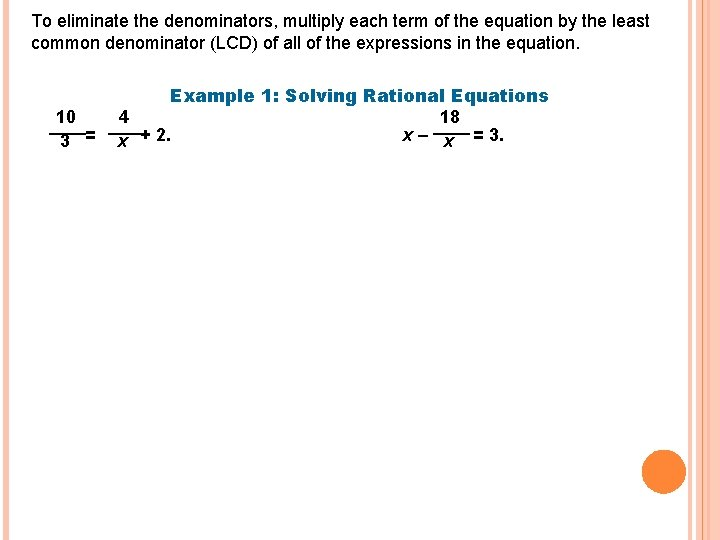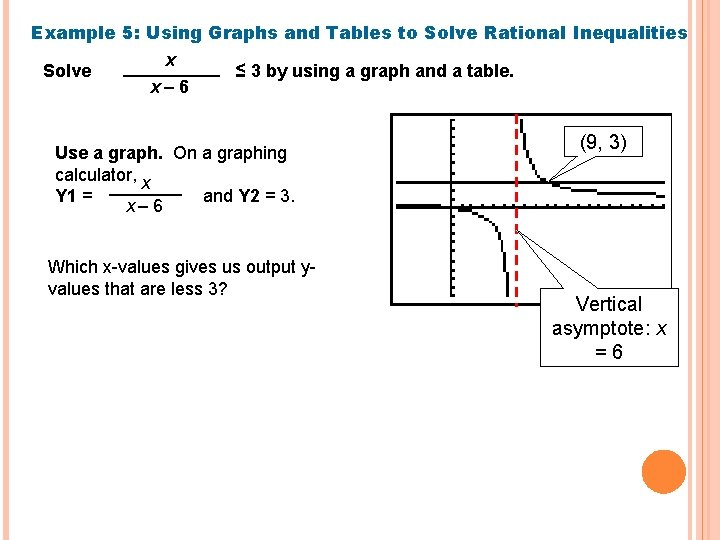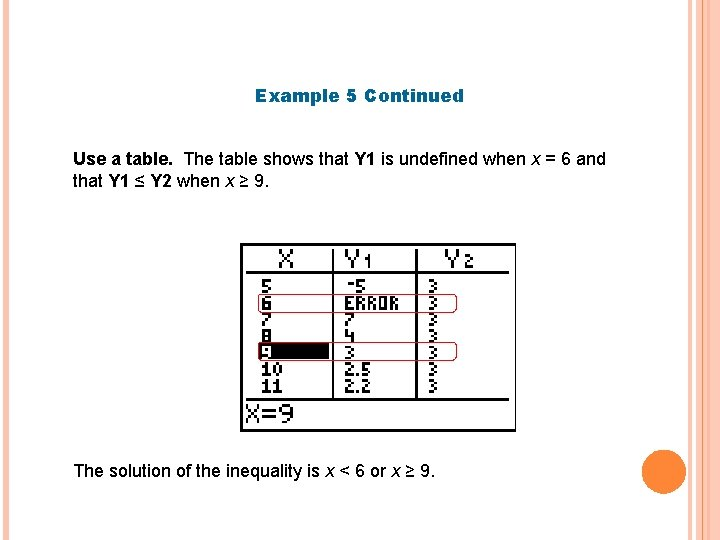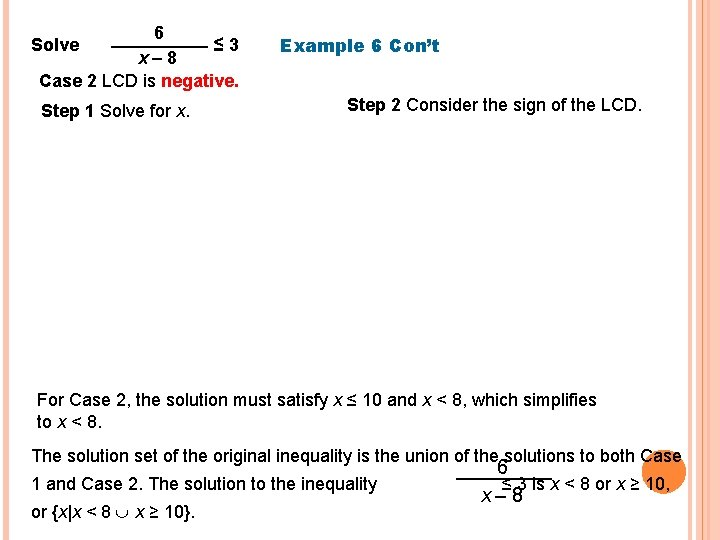# 8 5 SOLVING RATIONAL EQUATIONS AND INEQUALITIES Objective

• Slides: 78. 5 SOLVING RATIONAL EQUATIONS AND INEQUALITIES Objective Solve rational equations and inequalities. Vocabulary rational equation extraneous solution rational inequality aAlways check for extraneous solutions by substituting into the equation. Example 2 A: Extraneous Solutions Solve each equation. 5 x x– 2 Check: = 3 x + 4 x– 2 2 x – 5 x– 8 + x 2 = 11 x– 8To eliminate the denominators, multiply each term of the equation by the least common denominator (LCD) of all of the expressions in the equation. 10 3 = Example 1: Solving Rational Equations 4 18 x – x = 3. x + 2.Example 5: Using Graphs and Tables to Solve Rational Inequalities Solve x x– 6 ≤ 3 by using a graph and a table. Use a graph. On a graphing calculator, x Y 1 = and Y 2 = 3. x– 6 Which x-values gives us output yvalues that are less 3? (9, 3) Vertical asymptote: x =6Example 5 Continued Use a table. The table shows that Y 1 is undefined when x = 6 and that Y 1 ≤ Y 2 when x ≥ 9. The solution of the inequality is x < 6 or x ≥ 9.To solve rational inequalities algebraically multiply each term by the LCD of all the denominators. You must consider two cases: the LCD is positive or the LCD is negative. Example 6: Solving Rational Inequalities Algebraically 6 Solve ≤ 3 x– 8 Case 1 LCD is positive. Step 1 Solve for x. Step 2 Consider the sign of the LCD. For Case 1, the solution must satisfy x ≥ 10 and x > 8, which simplifies to x ≥ 10.6 ≤ 3 x– 8 Case 2 LCD is negative. Solve Step 1 Solve for x. Example 6 Con’t Step 2 Consider the sign of the LCD. For Case 2, the solution must satisfy x ≤ 10 and x < 8, which simplifies to x < 8. The solution set of the original inequality is the union of the solutions to both Case 1 and Case 2. The solution to the inequality or {x|x < 8 x ≥ 10}. 6 ≤ 3 is x < 8 or x ≥ 10, x– 8